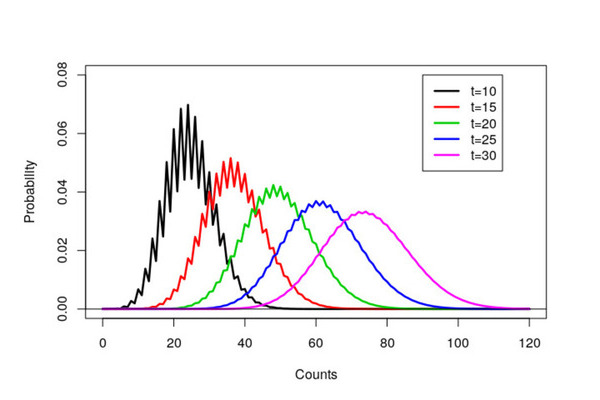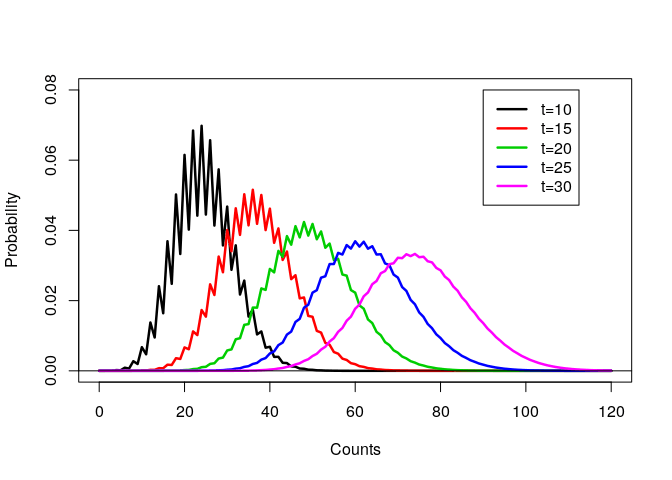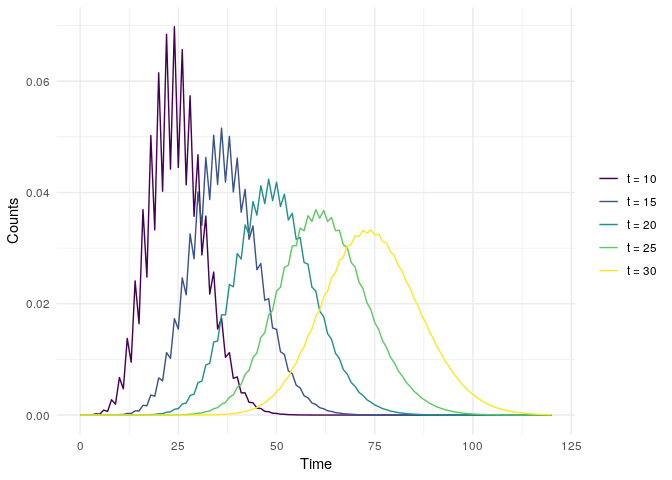GitHub

# R from the turn of the century

Published: September 20, 2018Last week I spent some time reminiscing about my PhD and looking through some old R code. This trip down memory lane led to some of my old R scripts that amazingly still run. My R scripts were fairly simple and just created a few graphs. However now that I’ve been programming in R for a while, with hindsight (and also things have changed), my original R code could be improved.

I wrote this code around April 2000. To put this into perspective,

• R mailing list was started in 1997
• R version 1.0 was released in Feb 29, 2000
• The initial release of Git was in 2005
• StackOverflow was launched in 2008

Basically, sharing code and getting help was much more tricky than today - so cut me some slack!

## The Original Code

My original code was fairly simple - a collection of `scan()` commands with some `plot()` and `lines()` function calls.

``````## Bad code, don't copy!
## Seriously, don't copy
par(cex=2)
a<-scan("data/time10.out",list(x=0))
c<-seq(0,120)
plot(c,a\$x,type='l',xlab="Counts",ylim=c(0,0.08),ylab="Probability",lwd=2.5)
a<-scan("data/time15.out",list(x=0))
lines(c,a\$x,col=2,lwd=2.5)
a<-scan("data/time20.out",list(x=0))
lines(c,a\$x,col=3,lwd=2.5)
a<-scan("data/time25.out",list(x=0))
lines(c,a\$x,col=4,lwd=2.5)
a<-scan("data/time30.out",list(x=0))
lines(c,a\$x,col=6,lwd=2.5)
abline(h=0)
legend(90,0.08,lty=c(1,1,1,1,1),lwd=2.5,col=c(1,2,3,4,6), c("t=10","t=15","t=20","t=25","t=30"))
``````

The resulting graph ended up in my thesis and a black and white version in a resulting paper. Notice that it took over eight years to get published! A combination of focusing on my thesis, very long review times (over a year) and that we sent the paper via snail mail to journals.## How I should have written the code

Within the code, there are a number of obvious improvements that could be made.

1. In 2000, it appears that I didn’t really see the need for formatting code. A few spaces around assignment arrows would be nice.
2. I could have been cleverer with my `par()` settings. See our recent blog post on styling base graphics.
3. My file extensions for the data sets weren’t great. For some reason, I used `.out` instead of `.csv`.
4. I used `scan()` to read in the data. It would be much nicer using `read.csv()`.
5. My variable names could be more informative, for example, avoiding `c` and `a`
6. Generating some of the vectors could be more succinct. For example
``````rep.int(1, 5) # instead of
c(1, 1, 1, 1, 1)
``````

and

``````0:120 # instead of
seq(0, 120)
``````

Overall, other than my use of `scan()`, the actual code would be remarkably similar.

## A tidyverse version

An interesting experiment is how the code structure differs using the {tidyverse}. The first step is to load the necessary packages

``````library("fs") # Overkill here
library("purrr") # Fancy for loops
library("dplyr") # Manipulation of data frames
library("ggplot2") # Plotting
``````

The actual tidyverse inspired code consists of three main section

• Read the data into a single data frame/tibble using `purrr::map_df()`
• Cleaning up the data frame using `mutate()` and `rename()`
• Plotting the data using {ggplot2}

The amount of code is similar in length

``````dir_ls(path = "data") %>% # list files
col_names = FALSE) %>% # read & combine files
mutate(Time = rep(0:120, 5)) %>% # Create Time column
rename("Counts" = "X1") %>% # Rename column
ggplot(aes(Time, Counts)) +
geom_line(aes(colour = filename)) +
theme_minimal() + # Nicer theme
scale_colour_viridis_d(labels = paste0("t = ", seq(10, 30, 5)),
name = NULL) # Change colours
``````

and gives a similar (but nicer) looking graph.## I lied about my code working

Everyone who uses R knows that there are two assignment operators: `<-` and `=`. These operators are (more or less, but not quite) equivalent. However, when R was first created, there was another assignment operator, the underscore `_`. My original code actually used the `_` as the assignment operator, i.e.

``````a_scan("data/time10.out",list(x=0))
``````

``````a<-scan("data/time10.out",list(x=0))
I can’t find `_` operator was finally removed from R, I seem to recall around 2005.